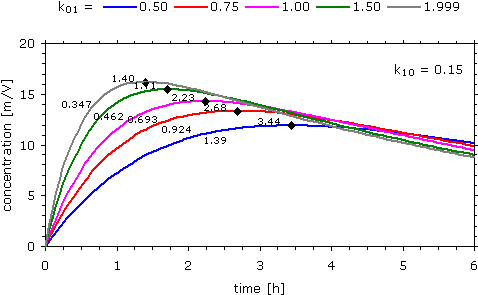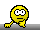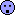BEAZ
★

India,
2008-07-11 13:35
(4763 d 10:22 ago)

Posting: # 2015
Views: 18,804

## Tmax and T1/2 [NCA / SHAM]

hai,
can any one clarify that, is there any relation between Tmax and t1/2. for some products the Tmax found to be very close to t1/2. how it is possible?
regards

Edit: Category changed. [Helmut]

Alhas
Helmut
★★★Vienna, Austria,
2008-07-11 17:08
(4763 d 06:49 ago)

@ BEAZ
Posting: # 2016
Views: 16,636

## Tmax and T½

Dear Alhas!

» can any one clarify that, …

Hopefully!» … is there any relation between Tmax and t1/2.

The regulatory definition of BE contains the phrase "… rate and extent of absorption …".
Unfortunately the rate-constant of absorption (different names: ka, kin, k01, …) often is difficult to estimate even with compartmental modeling. In NCA for a one-compartment model one can start with the Wagner-Nelson method. tmax is a rather insensitive metric of the rate of absorption (as shown by László Endrényi about 15 years ago). That’s the reason it’s only supportive (based on clinical grounds) in some regulations and partial AUCs are preferred by the FDA instead.
For an IR formulation the ‘slow’ phase kel, k10, …) is assumed to be the elimination of drug.
Let’s have a look at the simple one-compartment open model:$$C(t)=\frac{D\times F_{\textrm{a}}}{V_{\textrm{d}}}\times \frac{k_{01}}{k_{01}-k_{01}}\times\left (\exp (-k_{10}\times t)-\exp(-k_{01}\times t) \right ) \tag{1}$$We can calculate Cmax and tmax in the following way:
1. The slope of the function is positive before tmax (increasing values), zero at tmax, and negative (decreasing) afterwards.
2. Differentiate the function.
3. Find the root (i.e., the intersection of the derivative with the abscissa). This is tmax.
Formulas:$$t_{\textrm{max}}=\frac{1}{k_{01}-k_{10}}\times \log_{e}(k_{01}/k_{10}) \tag{2}$$and by substituting $$t$$ in $$(1)$$ with $$(2)$$ after some rearrangement (not shown)$$C_{\textrm{max}}=\frac{D\times F_{\textrm{a}}}{V_{\textrm{d}}}\times(k_{01}/k_{10})^{k_{10}/(k_{10}-k_{01})}\tag{3}$$Unfortunately it is not possible to differentiate functions of higher compartment models (more than two exponential terms). In other words, there’s no analytical solution – curve fitting programs approximate the solution by numeric integration of the differential equations by means of variants of the Gauss-Newton algorithm. So it’s nothing for M\$Excel (unless you trust in the ‘Solver’-Add-In – which you shouldn’t).

» for some products the Tmax found to be very close to t1/2. how it is possible?Chance?
I prepared an example:
D = 100
Vd = 5
Fa = 1
k01 = 2
k10 = 0.025 – 1.999

If the elimination is slow (k10 = 0.050) we get tmax 1.89 and t½ 13.9, if the elimination approaches the absorption (k10 = 1.999), we get tmax 0.500 and t½ 0.347…

Only for k10 = 1 we get tmax = t½ = 0.693 – which is loge(2)…

Therefore only for drugs with relatively fast elimination (as compared to absorption) tmax ~ t½;
$$t_\textrm{max}=t_{1/2}\; \leftrightarrow\; k_{10}=k_{01}/2\: \small{\square}$$For drugs with a biphasic elimination similar t½ and tmax are almost impossible.A further remark on the insensitivity of tmax to changes in the absorption rate: essentially the same example as above, but the elimination kept constant with k10 = 0.15 and the absorption varies between 0.50 and 1.999.

Setting a k01 of 1.0 as the reference value (tmax 2.23), a 10% change (k01 1.1) results in a 6% decrease in tmax (to 2.10).
If we increase k01 further by 50% to 1.5, tmax decreases only by 24% to 1.71.

That’s why we use tmax as a PK metric in BE only if unavoidable.Dif-tor heh smusma 🖖
Helmut SchützThe quality of responses received is directly proportional to the quality of the question asked. 🚮
Science Quotes
BEAZ
★

India,
2008-07-15 07:54
(4759 d 16:03 ago)

@ Helmut
Posting: # 2033
Views: 16,227

## Tmax and T1/2

Dear Helmut,
Thank you for the information.
still i am not clear.Tmax is the time reach the maximum concentration, logically after reaching a Tmax only the drug shall startes its elimination phase, if the T1/2 comes first then the conc of the drug in systemic circulation is reduced to its half and after this how we get a Tmaxkindly clarify
Thanks and Regards

Alhas
Ohlbe
★★★

France,
2008-07-15 10:33
(4759 d 13:24 ago)

@ BEAZ
Posting: # 2036
Views: 16,218

## Tmax and T1/2

Dear Alhas,

The body does not press a button at Tmax to stop absorbing the drug and to start eliminating it. Elimination starts at the second the drug enters the body. Tmax is the time when you reach a balance between absorption and elimination: before Tmax the drug enters the body faster than it is eliminated, and concentrations increase. After Tmax the drug is eliminated faster than it is absorbed, and concentrations decrease.

Regards
Ohlbe
Helmut
★★★Vienna, Austria,
2008-07-16 01:18
(4758 d 22:39 ago)

@ Ohlbe
Posting: # 2038
Views: 16,534

## Tmax and T1/2

Dear Ohlbe & Alhas!

» Elimination starts at the second the drug enters the body.

Exactly. There are many processes involved, all of them are stochastic ones. In PK we retreat into the concept of ‘compartments’, because we cannot better model our body consisting of 1013–1014 cells. Speaking of a one-compartment model, we treat blood and all highly perfused organs (liver, brain, kidneys; partly muscles) as a (well-stired) beaker with a small hole in the bottom. We assess these processes statistically; if we talk about the half life of two hours of a drug with a molecular mass of 200 g/mol after a bolus dose of 10 mg on the average 1.506×1019 molecules will have left the systemic circulation within the first two hours. Or modifying your example (∆ input of zero duration), about 2.9×1015 molecules leave the system in the first second!1 – or 962 ng if you prefer to weigh a bunch of molecules…
If we split the equation we can see that after an oral dose the elimination process itself is exactly the same as after a fast bolus (the concentration at t=0 is D/Vd, which I have set throughout the examples in this thread to 100/5).An nice property of the function is the intersection of the elimination with the profile at tmax/Cmax.
It’s clear why the starting point for the estimation of the half-life in the TTT-me­thod (see this post) was chosen.
Also one of Kamal Midha’s Mantras (‘Once absorption is over, formulation differences no longer apply.’)2 is obviously justified (valid for ≥2×tmax).

» Tmax is the time when you reach a balance between absorption and elimination: before Tmax the drug enters the body faster than it is eliminated, and concentrations increase. After Tmax the drug is eliminated faster than it is absorbed, and concentrations decrease.

Almost…In a one-compartment system both absorption and elimination are treated as constant. Therefore, there’s no faster/slower absorption/elimination throughout the profile. In the log-plot the parallel course after about 2×tmax is even more evident (in more mathematical terms after the inflection point of the first derivative; where the root of the first derivative = tmax).@Alhas:
I strongly suggest to study one of the basic textbooks on pharmacokinetics – otherwise it will be very difficult for you to design and evaluate BA/BE-studies. I’m not always in the mood to answer “neophytes”.
If you want a quick solution I recommend David Bourne’s A First Course in Pharmacokinetics and Biopharmaceutics.

1. Only in a Gedankenexperiment! Since the turn-around time of the circulation is about two minutes, it will take a little bit longer…
2. Midha KK, Hubbard JW, Rawson MJ. Retrospective evaluation of relative extent of absorption by the use of partial areas under plasma concentration versus time curves in bioequivalence studies on conventional release products. Europ J Pharm Sci 1996;4:381–4.

Dif-tor heh smusma 🖖
Helmut SchützThe quality of responses received is directly proportional to the quality of the question asked. 🚮
Science Quotes
BEAZ
★

India,
2008-07-24 14:09
(4750 d 09:48 ago)

@ Helmut
Posting: # 2078
Views: 16,029

## Tmax and T1/2

Dear Ohlbe & Helmut!
thanks a lot.

@Helmut:
» I strongly suggest to study one of the basic textbooks on pharmacokinetics – otherwise it will be very difficult for you to design and evaluate BA/BE-studies.» I’m not always in the mood to answer ‘neophytes’.» If you want a quick solution I recommend David Bourne’s A First Course in Pharmacokinetics and Biopharmaceutics.AlhasIng. Helmut Schütz